MATH 106  Calculus for Business and Economics   Fall, '00
Class Summaries/Outlines

 8/29 8/31 9/1 10/2 10/3 10/5 10/6 9/5 9/7 9/8 10/9 10/10 10/12 10/13 9/11 9/12 9/14 9/15 10/16 10/17 10/19 10/20 9/18 9/19 9/21 9/22 10/23 10/24 10/26 10/27 9/25 9/26 9/28 9/29 10/30 10/31 11/1 11/2

• 8/29
• Class spent much time on problems from the review sheet related to functions.

• Function notation was reviewed. Mapping(transformation) figures and graphs of functions were introduced .
2. For this problem let f be defined by f(x) = 5x2 + 3.
A. Find the following. Simplify you answer when possible.
i) f(1)  = 5(1)2 + 3=8                    iii) f(1+h)= 5(1+h)2 + 3= 8+10h+5h2
ii) f(h)  =  5h2 + 3        iv) [f(1+h)-f(1)]/h = [10h +5h2 ]/h=h[10+5h]/h= 10 +5h
B. Find any number(s) z where f(z) = 23.
f(z)= 5z2 + 3= 23 ;     5z2 =20;   ... z = 2  OR z = -2.
OR           5z - 20 = 0;  5(z+2)(z-2)=0; ... z = 2 OR z = -2.
C. For which values of x is f(x) < 23? Express your answer as an interval.
• We examined f using a table of selected values:

•
 x f(x)= 5x2  +3 3 48 2 23 1 8 0 3 -1 8 -2 23 -3 48

and a transformation (mapping) figure: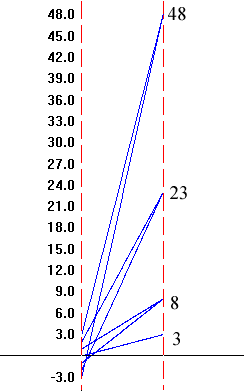Based on this  we concluded that the solution was {x | -2<x<2} = (-2,2).

After the analysis of the transformation figure we considered when x+2<0.... x< -2. We graphed this on a number line.

----------------0 +++++++++++++++  (x+2)
-2     0

Then we considered
f(x)= 5x2 + 3 < 23; so  5x - 20 < 0;   5(x+2)(x-2) < 0. We then scanned the line with information about the factors (x+2) and (x-2)  to investigate when the expression  5x - 20 would be positive, negative, or 0.

-------------------------0+++++++++   (x-2)
----------------0 +++++++++++++++  (x+2)
-2     0     2
+++++++++++0--------0+++++++++   5(x+2)(x-2)

Thus  -5x - 20 < 0 when 2<x<2... or x is in the interval (-2,2).

• In summary, we examined f using a table of selected values:

•
 x f(x)= 5x2  +3 3 48 2 23 1 8 0 3 -1 8 -2 23 -3 48

a transformation (mapping) figure:and finally a graph.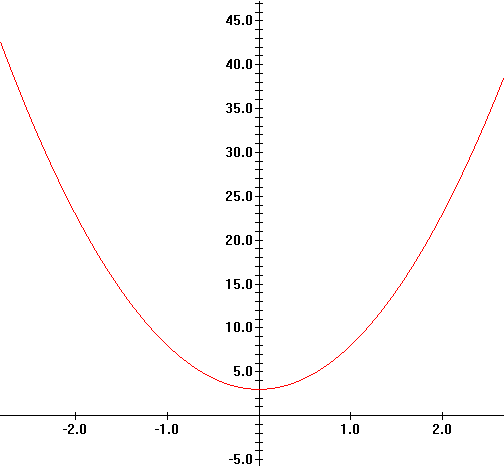• 8/31 ( and beyond!)

• Numbers, Variables, Algebra Review 1.1&1.2
• Go over Background Analysis.
• Solutions:
1. B: y=2x+5
2. D: -9/10
3. A:  (x-1)/x^3
4. D:  x^2/(3y^9)
5. D:  3x + 2 sqrt(15xy) + 5y
6. C:  \$52
7. D: 4
Summary of Chapter 1 in Calculus Applied to the Real World (on-line)
• SECTION 1.1
• Real numbers are decimals (possibly infinite and non-repeating)
• The number line. Points on the line correspond to real numbers.
• Absolute values.
• Example:  |-2|=2;  |sqrt(5)-5| = 5-sqrt(5).
• Example*:  |2 + - 3| < |2| + |-3|
• Equality, inequality, and intervals.
• Solving a linear equation.
• Example.  3x+5=29:

• 3x= 24;  x =8.... check.
• Solving a linear inequality.
• Example:   3x+5<29:

•   3x<24;    x<8...  x in the interval (-inf,8).
(No total check- but some confirming evidence!)
• Example:   -2x < 10:

•     x > -5 .... x in the interval (-5, inf).
• integer exponents:
• 2^3 = 2*2*2 = 8
• 2^0 = 1
• 2^-3 =1/ (2^3) = 1/8
• fraction exponents and roots
• 8^(1/3) = cube root of 8 = 2
• Properties:
• SAME BASE: Products, quotients, powers
• SAME POWER: Products. quotients.

•
• SECTION 1.2
• Multiplication and Factoring:
• a*(b+c)= a*b + a*c
• quadratic expressions (see text p 17)
• Roots and equations.
• Example:  x^2-5x + 6 = 0 :

•   Factor (x-3)*(x-2)= 0
so either x-3=0 or x-2 = 0.
Thus x=3 or x=2 are solutions. CHECK!
• Example:  x^2-5x + 6 = 0 :

• x=[-(-5)+ or- sqrt( (-5)^2-4*1*6)]/2*1
=[ 5 + or - sqrt(25-24)]/2
= [5 + or - 1]/2
= 6/2 or 4/2
so x = 3 or 2.
• Fractions  (Rational Expressions)
• Factor and "cancel"
• Common Denominators [Still to be reviewed.]
• Rationalizing expressions with roots. [Still to be reviewed.]

•
• SECTION 1.3
• Cartesian Coordiantes

•
• 9/1 Starts Here!
• MATH 046 [CRN: 43406] is an algebra review Workshop for ELM & MPT Review. It is .5 units and meets

• Friday Sept. 1  1900-2050 in SH 110 and
Saturday Sept. 2  0900-1550 SH 110.
This workshop should review much of what you will use in Math 106.
You will not need to take the test at the end of the workshop since you already have a code 40.

• Distance
• Example: eq'n of a circle with center at (1,2) and radius 3.

•
• SECTION  1.4 (some review here from last class)
• Slope
• m =  (y2- y1)/ (x2-x1)
• Example: find slope of line through the points (4, 2) and (1,3):

• slope= m = (3-2)/(1-4) = 1/(-3) = - 1/3
• Interpret: m>0; m<0; m=0
• parallel non-vertical lines have the same slope.
• Equations for lines:
• Vertical:
• Example:X=3
• Non-vertical:
•  Point- slope
• Example: m=5 through (2,3):

• Y-3=5(X-2)   or   Y=5(X-2)+3
•  slope- Y intercept
• Example: m=5 Y intercept is (0,7)

• Y=5X + 7
• General Equation:
• Example: find X and Y intercepts for 2X + 3Y - 6 = 0:

• Set X= 0;   3Y=6;   Y=2   so (0,2) is the Y intercept.
Set Y=0;    2X=6;   X=3  so (3,0) is the X intercept.
• 9/5 And 9/7 We did more on lines and ....
• SECTION 2.1   FUNCTIONS.
• What is a function?
• A function f is a correspondence (rule) which assigns to each member of the domain (source) set, x, a member of the co-domain (target) set, denoted f(x) .

• How are functions visualized?
• Mapping figures/transformation figures.

•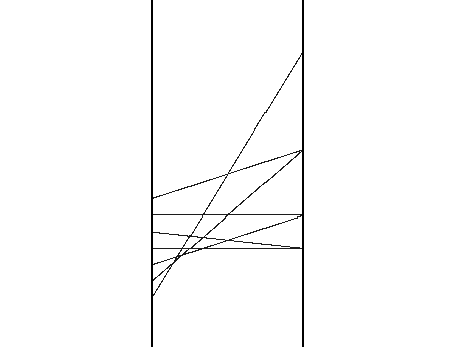• Graphs

• 9/8 starts here --
• How are functions defined?
• with a formula.

• The function g is defined by assigning to the number x the value x2+5. This is expressed by the equation: g(x)=x2+5.

With this definition we have
g(0)=5, g(1)=6, g(-1)=6,g(2)=9, g(1/2)=21/4, g(t)=t2+5, g(1+t)=(1+t)2+5, etc.

• with a table.

•
 x f(x) 2 5 1 3 0 -2 -1 4 -2 0
• with a graph.

•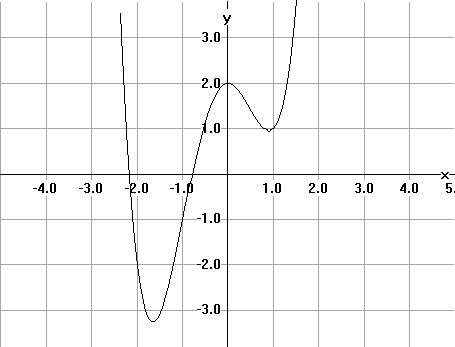The vertical line test: A graph measuring variables X and Y determines Y as a function of X if  each vertical line meets the graph at at most one point.
• with a context.
• Suppose that C is the cost of producing X liters of OURBEST wine. C is a function of X
• Problem #8:

•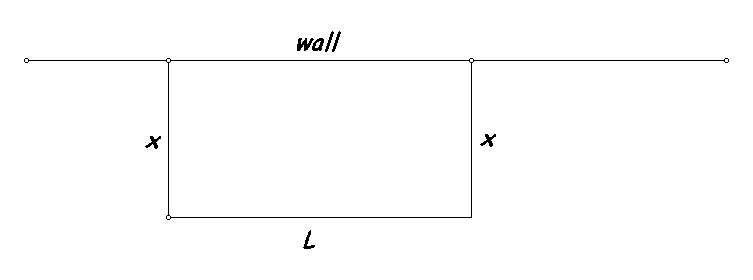The wall is 200 meters long. We have 140 meters of fence. This is a constraint. In terms of the lengths measured x and L we have that 2x+L = 140.  Thus L = 140 -2x.
The area, A, is found by multiplying x by L.  Thus A = x (140-2x) = 2x(70-x). We made a table of some values of this function.  We noted that 0<=x<=70.

 x L A 10 120 1200 20 100 2000 70 0 0 60 20 1200 50 40 2000
• We looked at the transformation figure from this data as well as the graph.

• This led to a conjecture from the symmetry that the largest value for the area, A, would occur when x = 35. in this case A=35*70=2450.

In fact, A = -2x2+140x  but A also can be expressed by A= -2(x-35)2 +2450. It should be clear from thinking about this expression for A that A is at most 2450, since for any x other than 35, A will be the result of subtracting a positive number from 2450.

• We then examined this function's graph using WINPLOT.
•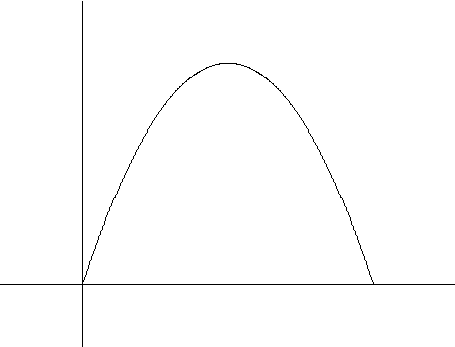• More on functions and Mathematical Modeling. (2.1 and 2.3)
Use Winplot to graph examples of these with parameters.
• More examples of functions.

• f(x)= Ax2 + Bx + C
• Functions defined in cases.

• i. s(x)= 2x+1 when x <3 ; -x+10 when x>=3
ii s(x)= 2x+1 when x <3 ; Ax+B when x>=3

• Polynomial functions.

• cubic c(x)= Ax3 +Bx 2 + Cx + D
quartics q(x)= Ax4 + Bx + Cx 2 +Dx +E

• Rational functions.

• R(x)=f(x)/g(x) where f and g are polynomials.
Examples: use polynomials above for f and g.

Example: Average cost= C(x)/x

• Power/root functions.

• examples: f(x)=sqrt(x); g(x)= x1/3

• A few details on functions- especially  considering equilibrium for supply/demand.
• Demand   p =f( xd)  N.B. This reverses the more sensible relationship as xd being a function of p.
• Supply   p =f( xs).  N.B. This also reverses the more sensible relationship as xs being a function of p.
•
Use Winplot to graph examples.